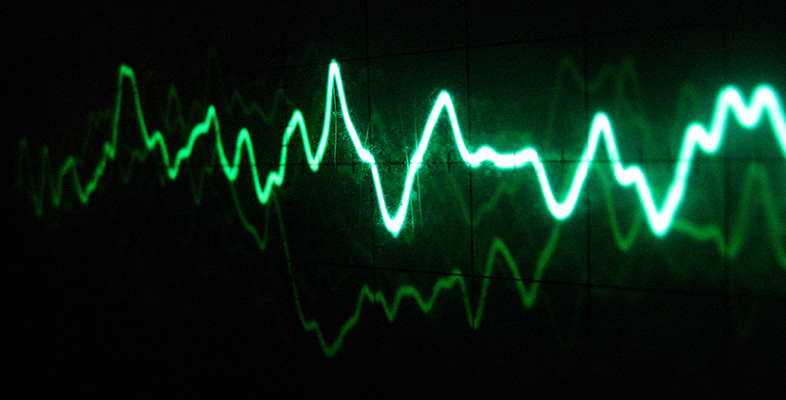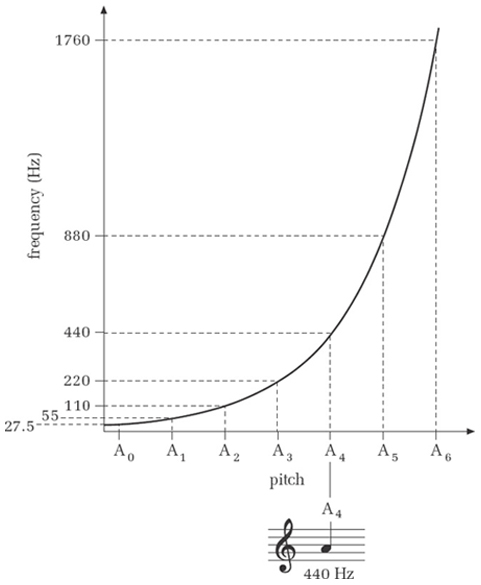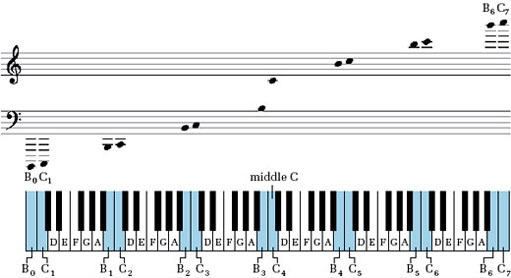### OU short coursesSound for music technology: An introduction

Start this free course now. Just create an account and sign in. Enrol and complete the course for a free statement of participation or digital badge if available.

# 8.2 Octave pitch and frequency increments

Because a doubling of frequency corresponds to an octave increase of pitch, it follows that there is no constant increment of frequency that always corresponds to a one-octave increment of pitch. That is to say, there is no fixed amount by which a frequency can be augmented that will always produce a one-octave pitch rise.

For instance, starting at the pitch A4 with a frequency of 440 Hz, we need to augment the frequency by 440 Hz to get the pitch one octave above (880 Hz). But a further augmentation of 440 Hz does not take us to the next A. We need an augmentation of 880 Hz to reach the next A. Clearly the frequency steps get bigger as the frequency (and pitch) get higher. In Figure 24, the horizontal axis shows a series of As an octave apart. Notice that they are equally spaced, corresponding to the equal pitch step of one octave between each. The vertical axis shows the frequency corresponding to each pitch. Notice that these frequencies are not equally spaced.Figure 24 Pitch/frequency graph

The same effect is found with any pair of pitches as we move up through the frequency range. For instance, the step from any C to the G above is five white notes on a piano keyboard, irrespective of whether you play it at the top end of the keyboard or at the bottom. In terms of named musical pitches, therefore, the step is always the same size: five notes. The size of the frequency step, however, is not at all constant, being much wider at the upper end of the keyboard than at the lower. What is constant is the ratio of the frequencies, being 1.5:1 for G and C (that is, the frequency of G is 1.5 times that of the first C below it, whatever the region of the keyboard). Similarly, the frequency step for a single tone, from C to the D above it for example, is not a fixed amount and varies from octave to octave; but the ratio of their frequencies is always the same. It is generally true that equal increments of musical pitch correspond to equal ratios of frequency, not equal increments of frequency.

You will have noticed that I have used the subscript convention for octave ranges. However, there are a number of alternative conventions in use that you may come across outside this unit. As a reminder, Box 3 restates the subscript convention, but also mentions two other systems.

## Box 3: Typographical notations for octave ranges

Several typographical systems have been devised for indicating the octave range in which a particular pitch is situated. A common convention, and the one used in the course, uses numerical subscripts. In this convention, each new octave is regarded as starting on C and ending on the B above, and the lowest range is given the subscript 0. Figure 25 shows a keyboard and a notational representation of this convention.Figure 25 Subscript system for indicating pitch regions

Another system you might come across is the so-called Helmholtz system. In this system C″ stands for C0, C′ for C1, C for C2, c for C3, c′ for C4, c″ for C5, etc.

Yet another system uses CCCC for C0, CCC for C1, CC for C2, C for C3, c for C4, c′ for C5, c″ for C6, etc.

## Activity 26 (Self-Assessment)

Suppose the keys on a piano keyboard were spaced in proportion to their frequency.

• (a) At which end of the keyboard would the keys be closer together?

• (b) On a four-octave keyboard where the bottom octave occupied 20 centimetres, what would be the total width of the keyboard?